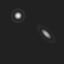## planetarium

Sub-pixel precision light spot rendering library

### 8 releases

 0.2.0 Mar 16, 2023 Mar 13, 2023 Apr 30, 2022 Mar 3, 2022 Nov 24, 2021

#31 in Science

Used in pyplanetarium

81KB
1.5K SLoC

# Planetarium

Sub-pixel precision light spot rendering library for astronomy and video tracking applications.

## Sample image## Example usage

``````use planetarium::{Canvas, SpotShape};

// Draw on a square 256x256 pixel canvas.
let mut c = Canvas::new(256, 256);

// Define a round spot shape with diffraction radius of 2.5 pixels.
let shape = SpotShape::default().scale(2.5);

// Add some spots at random positions with varying shape size
// and peak intensity.
let spot1 = c.add_spot((100.3, 130.8), shape, 0.5);
let spot2 = c.add_spot((80.6, 200.2), shape.scale(0.5), 0.9);

// Note: Out of range position coordinates and peak intensities are fine.
//       The resulting spot image is clipped into the canvas rectangle.
//       Peak intensity > 1.0 leads to saturation to the maximum pixel value.
let spot3 = c.add_spot((256.1, 3.5), shape.scale(10.0), 1.1);

// Set the canvas background pixel value.
c.set_background(100);

// Clear the canvas and paint the light spots.
c.draw();

// Access the rendered image data as a linear pixel array.
let image_pixbuf = c.pixels();

// Get pixel at x = 100, y = 200.
let (x, y) = (100, 200);
let (image_width, image_height) = c.dimensions();
let val_x_y = image_pixbuf[(y * image_width + x) as usize];
``````

Some of the light spot parameters like coordinates and peak intensity can be adjusted after the spot has been added to the canvas.

The spot position coordinates can be changed by adding an offset vector and the peak intensity can be adjusted by multiplying with a spot illumination factor.

It is possible to define a custom world coordinates to canvas coordinates transformation, which affects all light spots.

``````use planetarium::{Canvas, SpotShape, Transform};

// Draw on a square 256x256 pixel canvas.
let mut c = Canvas::new(256, 256);

// Define an elliptic spot shape with diffraction radii of 2.5 x 1.5 pixels
// rotated by 45 degrees counter-clockwise.
let shape1 = SpotShape::default().stretch(2.5, 1.5).rotate(45.0);

// Define an elliptic spot shape by a 2x2 linear transform matrix.
let shape2 = SpotShape::from([[2.0, -0.5], [1.5, 3.0]]);

// Add some spots at random positions with varying shape size
// and peak intensity.
let spot1 = c.add_spot((100.3, 130.8), shape1, 0.5);
let spot2 = c.add_spot((80.6, 200.2), shape2, 0.9);

// Shift the rendered spot positions by applying the relative offset vectors.
// The intrinsic spot position coordinates are immutable.
c.set_spot_offset(spot1, (-34.2, 12.6));
c.set_spot_offset(spot2, (114.2, -73.3));

// Adjust the rendered spot peak intensity by applying the spot illumination factors.
// The intrinsic spot intensities are immutable.
c.set_spot_illumination(spot1, 1.2);
c.set_spot_illumination(spot2, 0.7);

// Query the resulting spot coordinates on the canvas.
let pos1 = c.spot_position(spot1).unwrap();
let pos2 = c.spot_position(spot2).unwrap();

// Query the resulting peak spot intensities.
let int1 = c.spot_intensity(spot1).unwrap();
let int2 = c.spot_intensity(spot2).unwrap();

// Apply a custom world coordinates to canvas coordinates transformation.
c.set_view_transform(Transform::default().translate((13.7, -20.3)));

// Query the resulting spot coordinates on the canvas after
// the view coordinate transformation.
let pos1x = c.spot_position(spot1).unwrap();
let pos2x = c.spot_position(spot2).unwrap();
``````

## Canvas image export

The `Canvas` object supports image export to RAW and PNG file formats. Both 8-bit and 16-bit PNG sample formats are supported. Export to PNG formats requires the default `png` feature to be enabled.

### Example image export code

``````let mut c = Canvas::new(256, 256);

c.set_background(1000);
c.clear();

// Export to a 8-bit gamma-compressed grayscale RAW image.
let raw_8bpp_bytes = c.export_image(ImageFormat::RawGamma8Bpp).unwrap();

// Export to a 10-bit linear light grayscale little-endian RAW image.
let raw_10bpp_bytes = c.export_image(ImageFormat::RawLinear10BppLE).unwrap();

// Export to a 12-bit gamma-compressed grayscale little-endian RAW image.
let raw_12bpp_bytes = c.export_image(ImageFormat::RawLinear12BppLE).unwrap();

// Export to a 8-bit gamma-compressed grayscale PNG image.
let png_8bpp_bytes = c.export_image(ImageFormat::PngGamma8Bpp).unwrap();

// Export to a 16-bit linear light grayscale PNG image.
let png_16bpp_bytes = c.export_image(ImageFormat::PngLinear16Bpp).unwrap();
``````

## Window image export

The `Canvas` object additionally supports windowed image export.

A single rectangular window represents a region of interest (ROI) on the canvas image. Window rectangle coordinates are represented by the public `Window` structure.

### Example window image export code

``````let c = Canvas::new(256, 256);

// Create a 32x16 pixels window with origin at (100, 150).
let wnd = Window::new(32, 16).at(100, 150);

let fmt = ImageFormat::RawGamma8Bpp;

// Export to the canvas window image bytes.
let raw_window_bytes = c.export_window_image(wnd, fmt).unwrap();
``````

## Subsampled image export

The `Canvas` object additionally supports subsampled image export with independent row and column subsampling factors.

Only whole canvas images can be exported with subsampling.

### Example subsampled image export code

``````let c = Canvas::new(256, 256);

let fmt = ImageFormat::RawLinear10BppLE;

// Column (X) and row (Y) subsampling factors
let factors = (4, 2);

// Export to the subsampled canvas image bytes.
let raw_sub_bytes = c.export_subsampled_image(factors, fmt).unwrap();
``````

~1.5MB
~28K SLoC